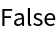#Function Repository Resource:

# ConnectedWolframModelQ

Determine whether a Wolfram model rule is left-connected

Contributed by: Max Piskunov
 ResourceFunction["ConnectedWolframModelQ"][rule] yields True if the left-hand side of the rule is connected, and the right-hand side is connected to the left-hand side. ResourceFunction["ConnectedWolframModelQ"][rule,All] yields True if both sides of the rule are separately connected, and also connected to each other.

## Details and Options

ResourceFunction["ConnectedWolframModelQ"][rule] yields True if the rule is local (left-hand side is connected). It may produce disconnected components, which it would not do if the right-hand side was connected to the left-hand side.
ResourceFunction["ConnectedWolframModelQ"][rule,All] also tests whether the right-hand side is connected. The rule can still however produce disconnected components even in ResourceFunction["ConnectedWolframModelQ"][rule,All].

## Examples

### Basic Examples (2)

An example of a connected rule:

 In:=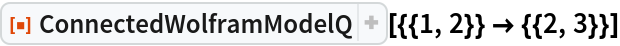Out=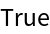An example of a disconnected rule:

 In:=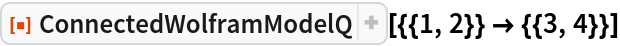Out=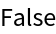### Scope (6)

Multiple rules are supported:

 In:=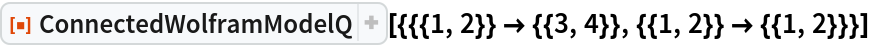Out=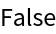In:=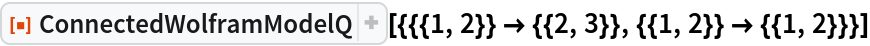Out=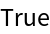Unary edges are supported:

 In:=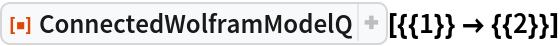Out=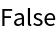In:=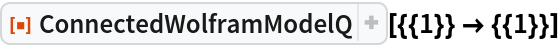Out=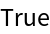In:=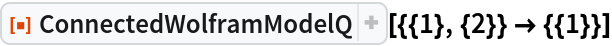Out=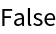By default, the right-hand side is allowed to be disconnected:

 In:=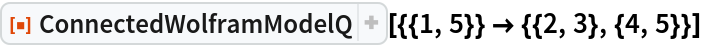Out=Left- and right- hand sides should be connected to each other:

 In:=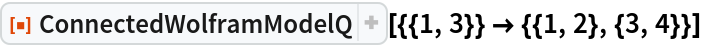Out=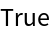While the right-hand side can be disconnected, each piece must be connected to the left-hand side:

 In:=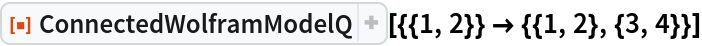Out=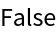One can also require the right-hand side to be connected separately:

 In:=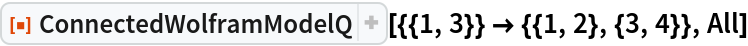Out=